HOME PAGE --------------- HELP+ » Grammar Notes --- » Ask a question » About EN Grammar » Phonetics --- » Nouns » Articles » Adjectives » Pronouns » Numerals » Verbs » Adverbs » Prepositions » Conjunctions » Interjections --- » Sentence Syntax » C_ S_ Syntax » Seq_ of Tenses » Inter_ & Negations --- » Punctuation » D/I Style ---------------# GRAMMAR NOTES: NUMERALS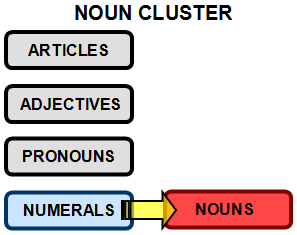Amazingly, despite the fact that using (complex) numerals is a direct meter of an advanced Civilization—therefore numerals are extremely important—most English grammar books do not trouble to consider numerals as forming a perfectly defined grammatical category (a morphologic element per se, same as the noun, for example). Most grammar authors label numerals as “adjectives”, “adverbs”, or [the easiest one] “quantifiers”, and they solve all problems by not presenting numerals at all!

In
LSEG4, on the other hand, numerals are presented according to their capital importance in Grammar. No other book in the entire World  comes close to LSEG4 when it comes to detailing numerals appropriately.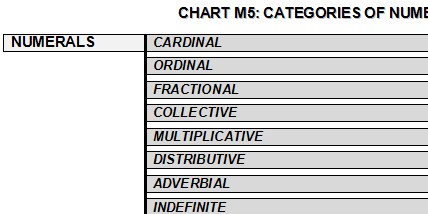It is worth mentioning the category of numerals named “indefinite numerals”. Many of them take articles, since all numerals are nouns, before anything elsedespite the fact that these indefinite numerals work, in most instances, as adjectives and adverbs (“the/a few”, “the/a little”).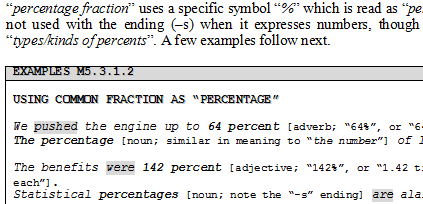Fragment from LSEG4: using Common Fraction (and the percentage numerals).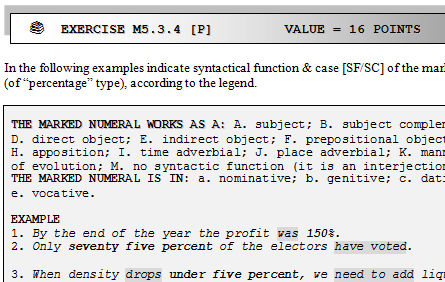Fragment from L4EW: exercises of analyzing  numerals' syntactic status in context.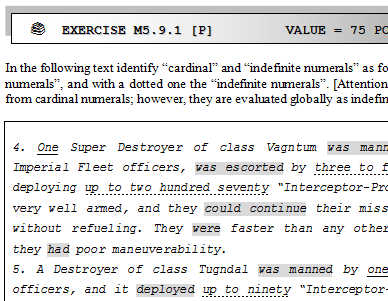Fragment from L4EW: identifying numerals in context.

INTERNATIONAL SYSTEM MKS [METER KELVIN SECOND]: STANDARD NUMERICAL PREFIXES [
excerpt from LSEG4 appendix "Technical Numerals"]

1 yocto [y] = 0.000000000000000000000001 = 10-24
1 zepto [z] = 0.000000000000000000001 = 10-21
1 atto [a]   = 0.000000000000000001 = 10-18
1 femto [f]  = 0.000000000000001 = 10-15
1 pico [p]   = 0.000000000001 = 10-12
1 nano [n]  = 0.000000001 = 10-9
1 micro [μ] / [u] = 0.000001 = 10-6
1 mili [m]    = 0.001 = 10-3
1 centi [c]  = 0.01 = 10-2
1 deci [d]   = 0.1 = 10-1

1 Deca/Deka [D] = 10 = 101
1 Hecto [H] = 100 = 102
1 Kilo [K]    = 1000 = 103
1 Mega [M] = 1000000 = 106
1 Giga [G]   = 1000000000 = 109
1 Terra [T]  = 1000000000000 = 1012
1 Peta [P]   = 1000000000000000 = 1015
1 Exa [E]    = 1000000000000000000 = 1018
1 Zetta [Z] = 1000000000000000000000 = 1021
1 Yotta [Y] = 1000000000000000000000000 = 1024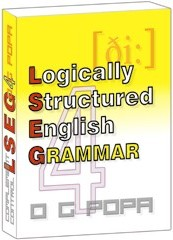A complete grammatical reference, very easy to learn: Logically Structured English Grammar 4—as theory plus exercises!Page last updated on: October 08, 2018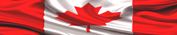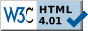Site pages valid according to W3C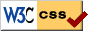Stylesheets pages valid according to W3C